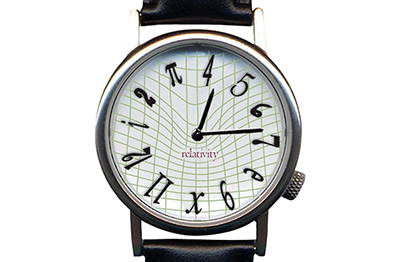# Watches are so much fun

Geometry Level 2The minute hand of a watch is $x$ times bigger than its hour hand. Let the area swept by the minute hand be $S_{m}$ and the area swept by the hour hand be $S_{h}$. Knowing the watch is perfectly adjusted and considering a fixed interval of time, the quotient of $\dfrac{S_{m}}{S_{h}}$ can be written as $kx^{2}$. Find $k$.

×